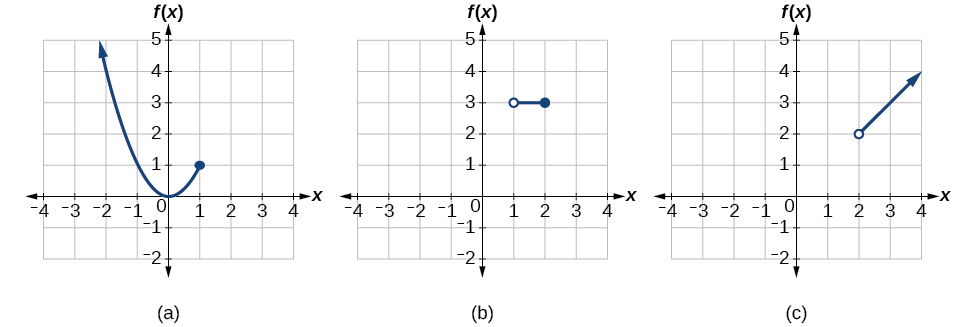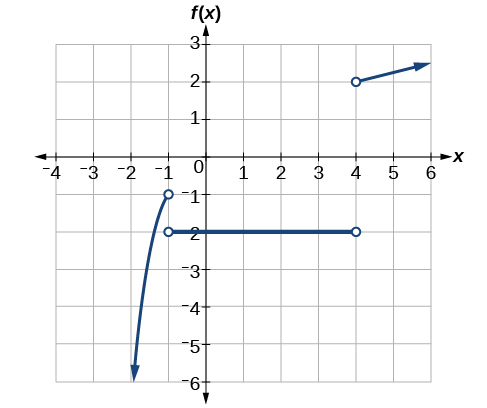# 3.2 Domain and range  (Page 7/11)

 Page 7 / 11

## Writing a piecewise function

A museum charges $5 per person for a guided tour with a group of 1 to 9 people or a fixed$50 fee for a group of 10 or more people. Write a function    relating the number of people, $\text{\hspace{0.17em}}n,\text{\hspace{0.17em}}$ to the cost, $\text{\hspace{0.17em}}C.$

Two different formulas will be needed. For n -values under 10, $\text{\hspace{0.17em}}C=5n.\text{\hspace{0.17em}}$ For values of $\text{\hspace{0.17em}}n\text{\hspace{0.17em}}$ that are 10 or greater, $\text{\hspace{0.17em}}C=50.$

$C\left(n\right)=\left\{\begin{array}{ccc}5n& \text{if}& 0

## Working with a piecewise function

A cell phone company uses the function below to determine the cost, $\text{\hspace{0.17em}}C,\text{\hspace{0.17em}}$ in dollars for $\text{\hspace{0.17em}}g\text{\hspace{0.17em}}$ gigabytes of data transfer.

$C\left(g\right)=\left\{\begin{array}{ccc}25& \text{if}& 0

Find the cost of using 1.5 gigabytes of data and the cost of using 4 gigabytes of data.

To find the cost of using 1.5 gigabytes of data, $\text{\hspace{0.17em}}C\left(1.5\right),\text{\hspace{0.17em}}$ we first look to see which part of the domain our input falls in. Because 1.5 is less than 2, we use the first formula.

$C\left(1.5\right)=\text{}25$

To find the cost of using 4 gigabytes of data, $\text{\hspace{0.17em}}C\left(4\right),\text{\hspace{0.17em}}$ we see that our input of 4 is greater than 2, so we use the second formula.

$C\left(4\right)=25+10\left(4-2\right)=\text{}45$

Given a piecewise function, sketch a graph.

1. Indicate on the x -axis the boundaries defined by the intervals on each piece of the domain.
2. For each piece of the domain, graph on that interval using the corresponding equation pertaining to that piece. Do not graph two functions over one interval because it would violate the criteria of a function.

## Graphing a piecewise function

Sketch a graph of the function.

$f\left(x\right)=\left\{\begin{array}{ccc}{x}^{2}& \text{if}& x\le 1\\ 3& \text{if}& 12\end{array}$

Each of the component functions is from our library of toolkit functions, so we know their shapes. We can imagine graphing each function and then limiting the graph to the indicated domain. At the endpoints of the domain, we draw open circles to indicate where the endpoint is not included because of a less-than or greater-than inequality; we draw a closed circle where the endpoint is included because of a less-than-or-equal-to or greater-than-or-equal-to inequality.

[link] shows the three components of the piecewise function graphed on separate coordinate systems.(a)   f ( x ) = x 2  if   x ≤ 1 ;   (b)   f ( x ) = 3  if 1<  x ≤ 2 ;   (c)   f ( x ) = x   if  x > 2

Now that we have sketched each piece individually, we combine them in the same coordinate plane. See [link] .

Graph the following piecewise function.

$f\left(x\right)=\left\{\begin{array}{ccc}{x}^{3}& \text{if}& x<-1\\ -2& \text{if}& -14\end{array}$Can more than one formula from a piecewise function be applied to a value in the domain?

No. Each value corresponds to one equation in a piecewise formula.

Access these online resources for additional instruction and practice with domain and range.

## Key concepts

• The domain of a function includes all real input values that would not cause us to attempt an undefined mathematical operation, such as dividing by zero or taking the square root of a negative number.
• The domain of a function can be determined by listing the input values of a set of ordered pairs. See [link] .
• The domain of a function can also be determined by identifying the input values of a function written as an equation. See [link] , [link] , and [link] .
• Interval values represented on a number line can be described using inequality notation, set-builder notation, and interval notation. See [link] .
• For many functions, the domain and range can be determined from a graph. See [link] and [link] .
• An understanding of toolkit functions can be used to find the domain and range of related functions. See [link] , [link] , and [link] .
• A piecewise function is described by more than one formula. See [link] and [link] .
• A piecewise function can be graphed using each algebraic formula on its assigned subdomain. See [link] .

the third and the seventh terms of a G.P are 81 and 16, find the first and fifth terms.
if a=3, b =4 and c=5 find the six trigonometric value sin
pls how do I factorize x⁴+x³-7x²-x+6=0
in a function the input value is called
how do I test for values on the number line
if a=4 b=4 then a+b=
a+b+2ab
Kin
commulative principle
a+b= 4+4=8
Mimi
If a=4 and b=4 then we add the value of a and b i.e a+b=4+4=8.
Tariq
what are examples of natural number
an equation for the line that goes through the point (-1,12) and has a slope of 2,3
3y=-9x+25
Ishaq
show that the set of natural numberdoes not from agroup with addition or multiplication butit forms aseni group with respect toaaddition as well as multiplication
x^20+x^15+x^10+x^5/x^2+1
evaluate each algebraic expression. 2x+×_2 if ×=5
if the ratio of the root of ax+bx+c =0, show that (m+1)^2 ac =b^2m
By the definition, is such that 0!=1.why?
(1+cosA+IsinA)(1+cosB+isinB)/(cos@+isin@)(cos$+isin$)
hatdog
Mark
jaks
Ryan
how we can draw three triangles of distinctly different shapes. All the angles will be cutt off each triangle and placed side by side with vertices touching

#### Get Jobilize Job Search Mobile App in your pocket Now!ByByBy Danielle StephensBy Briana KnowltonByBy Brooke DelaneyBy Ali SidBy Jonathan LongBy Tess ArmstrongByBy Janet ForresterBy OpenStax